# Class 12 Work sheet increasing and decreasing functions

5719

Mathematics Integration Level: Misc Level

Show that the function f(x) = 7x -3 is strictly increasing on R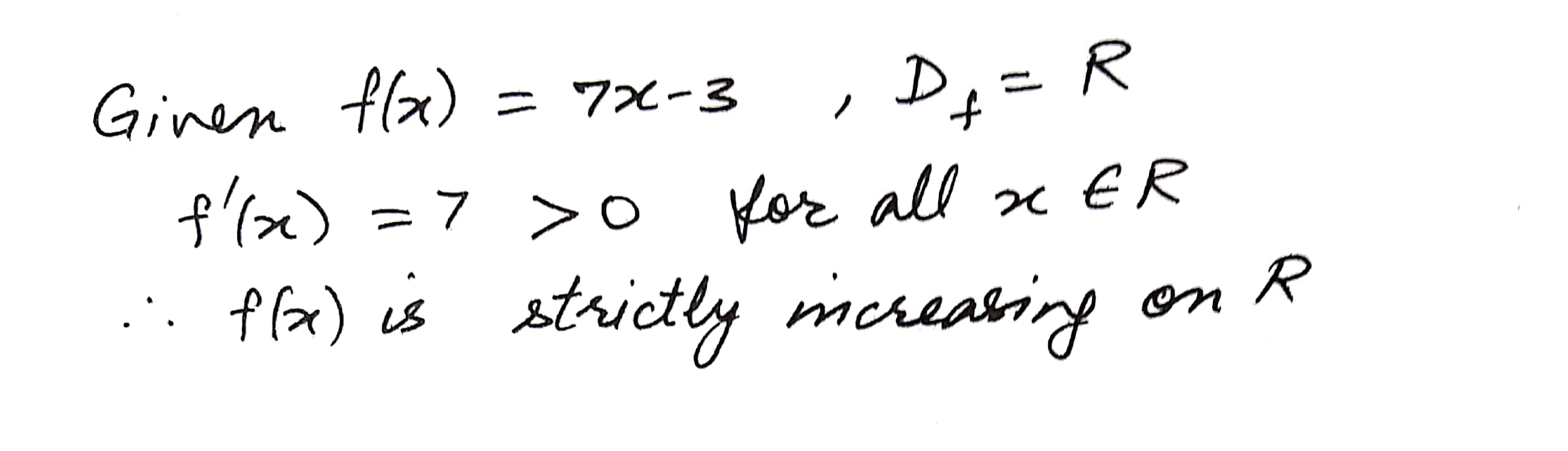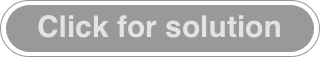5721

Mathematics Integration Level: Misc Level

Show that the function f(x) = log x is strictly increasing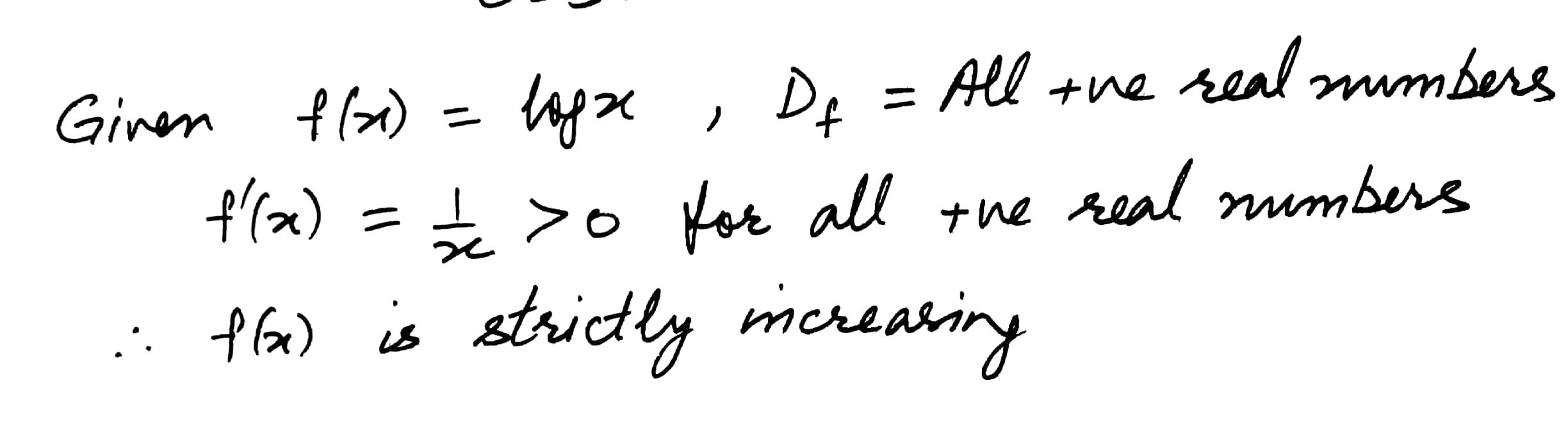5722

Mathematics Integration Level: Misc Level

Show that the function f given by f(x) = x3 - 3x2 + 4x, xR, is strictly increasing on R5723

Mathematics Integration Level: Misc Level

Prove that the function f given by f(x) = log Cos x is strictly decreasing on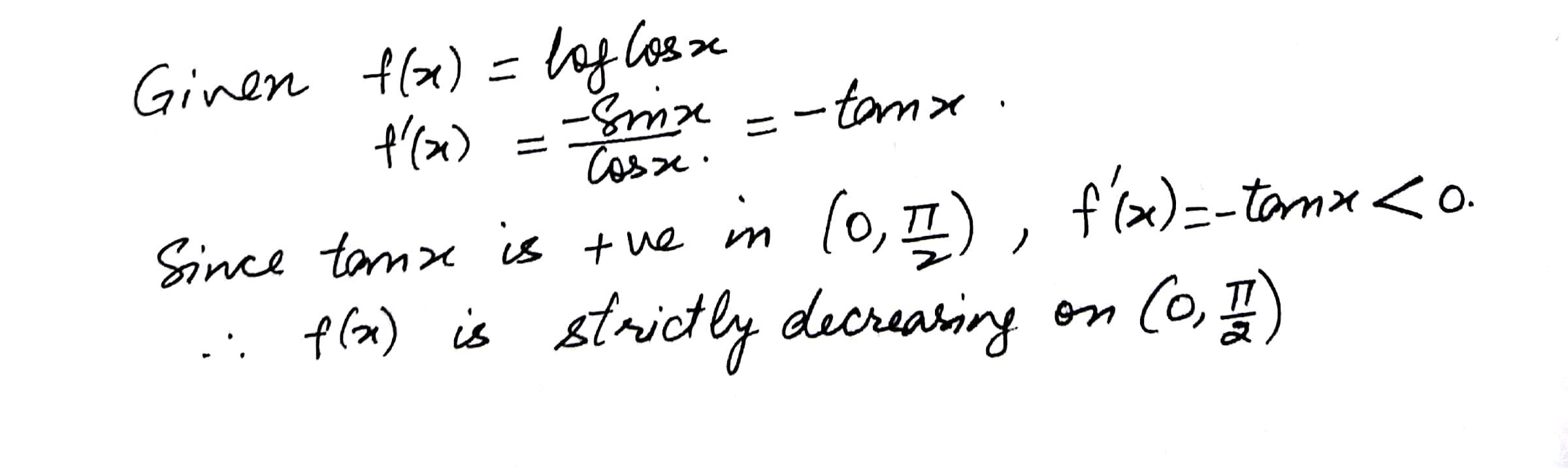5724

Mathematics Integration Level: Misc Level

Prove that the function f given by f(x) = Sin x is

(i)  strictly increasing on

(ii)  strictly decreasing on

(iii)  neither increasing nor decreasing in (0,)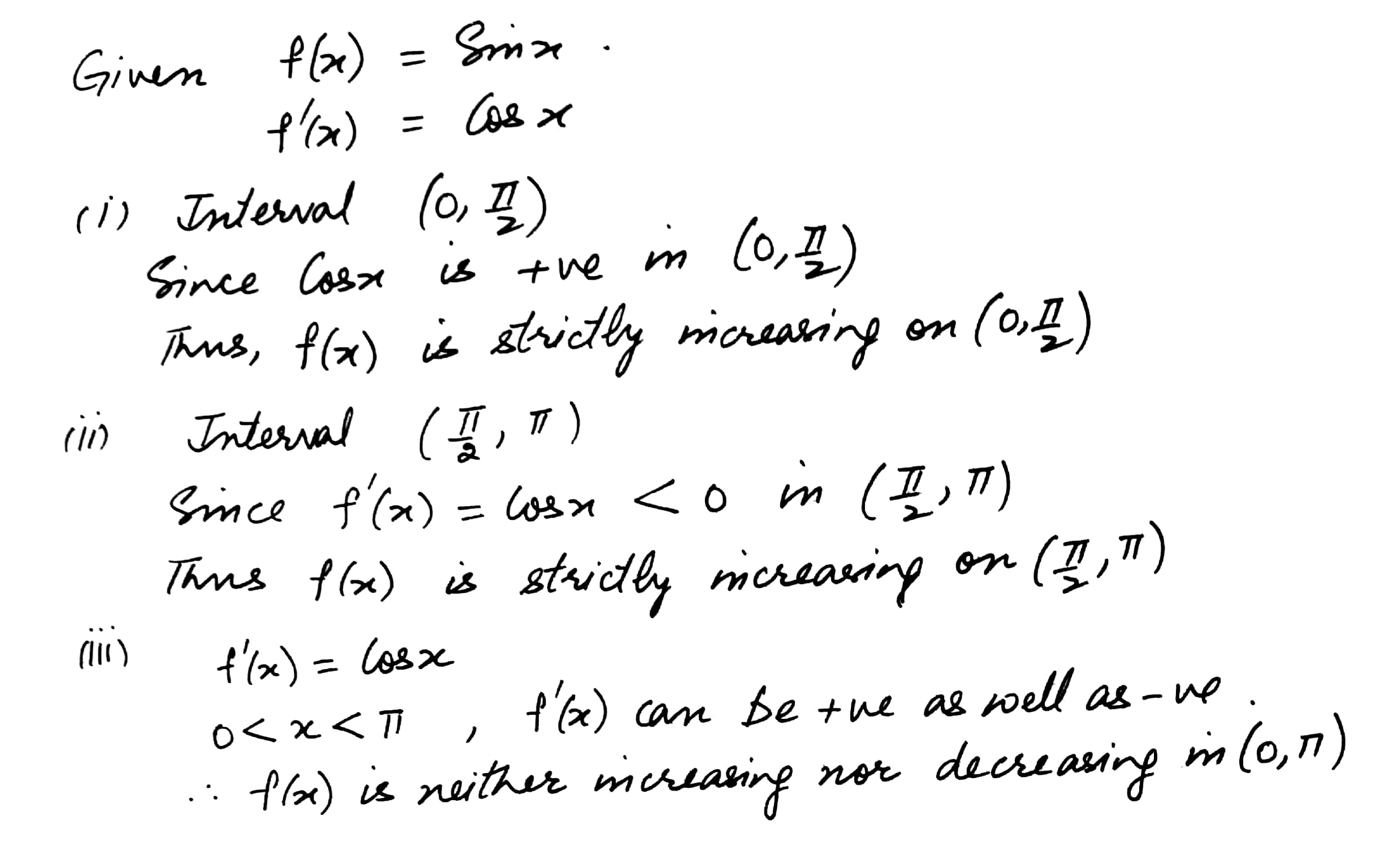5725

Mathematics Integration Level: Misc Level

Prove that the function f given by is increasing in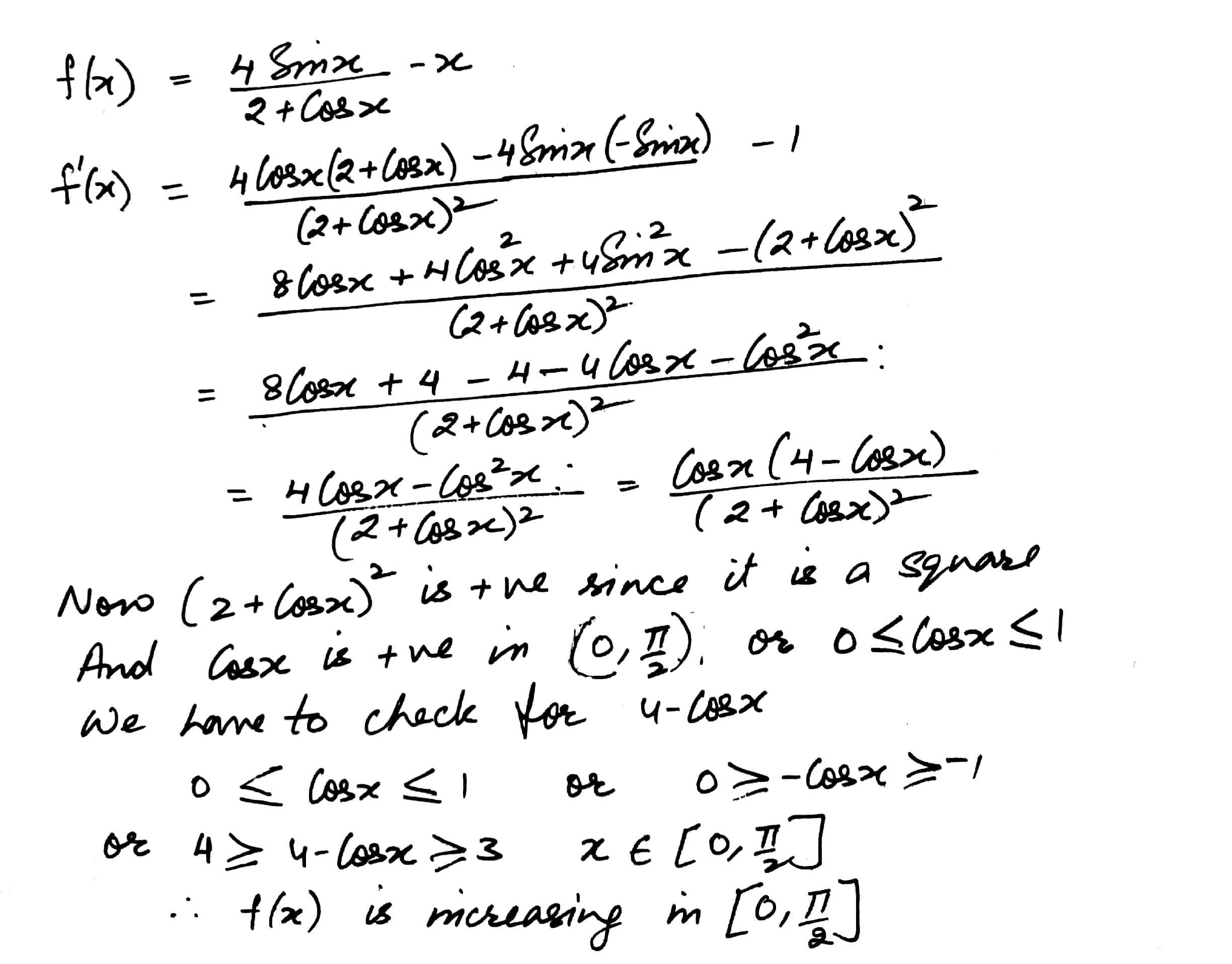5726

Mathematics Integration Level: Misc Level

Find the interval in which the following function is strictly increasing or strictly decreasing

f(x) = x2 - 4x + 6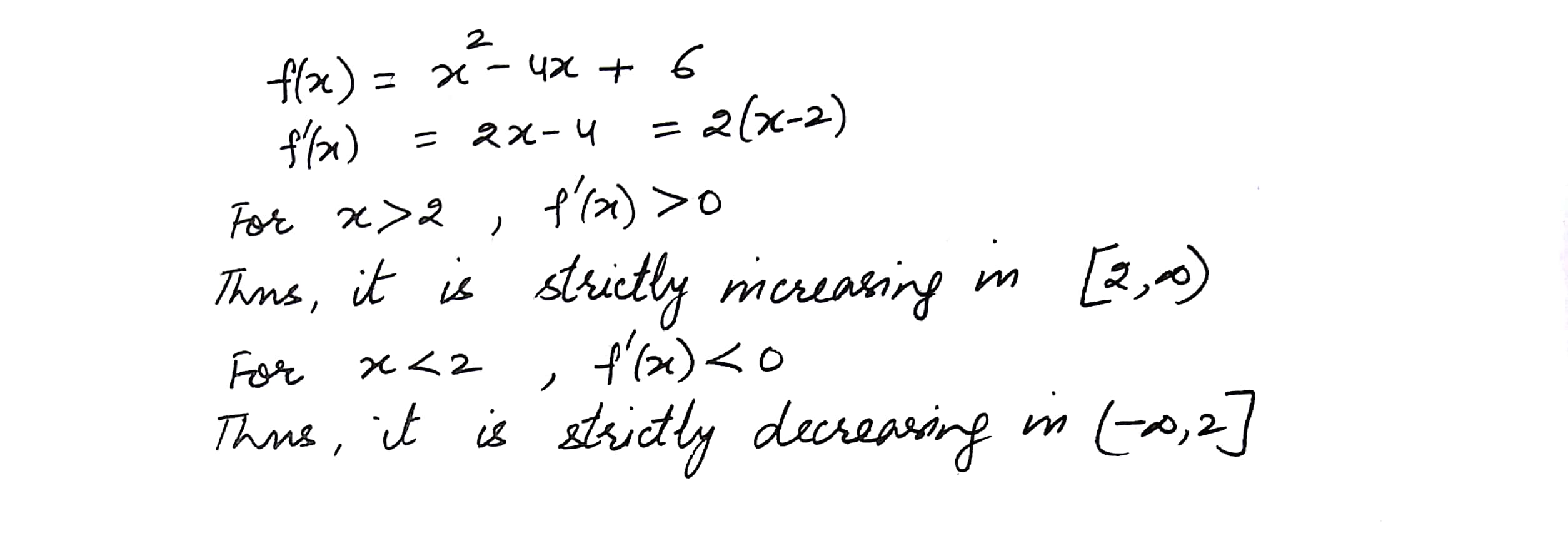5727

Mathematics Integration Level: Misc Level

Find the interval in which the following function is strictly increasing or strictly decreasing

f(x) = 2x3 - 3x2 - 36x + 7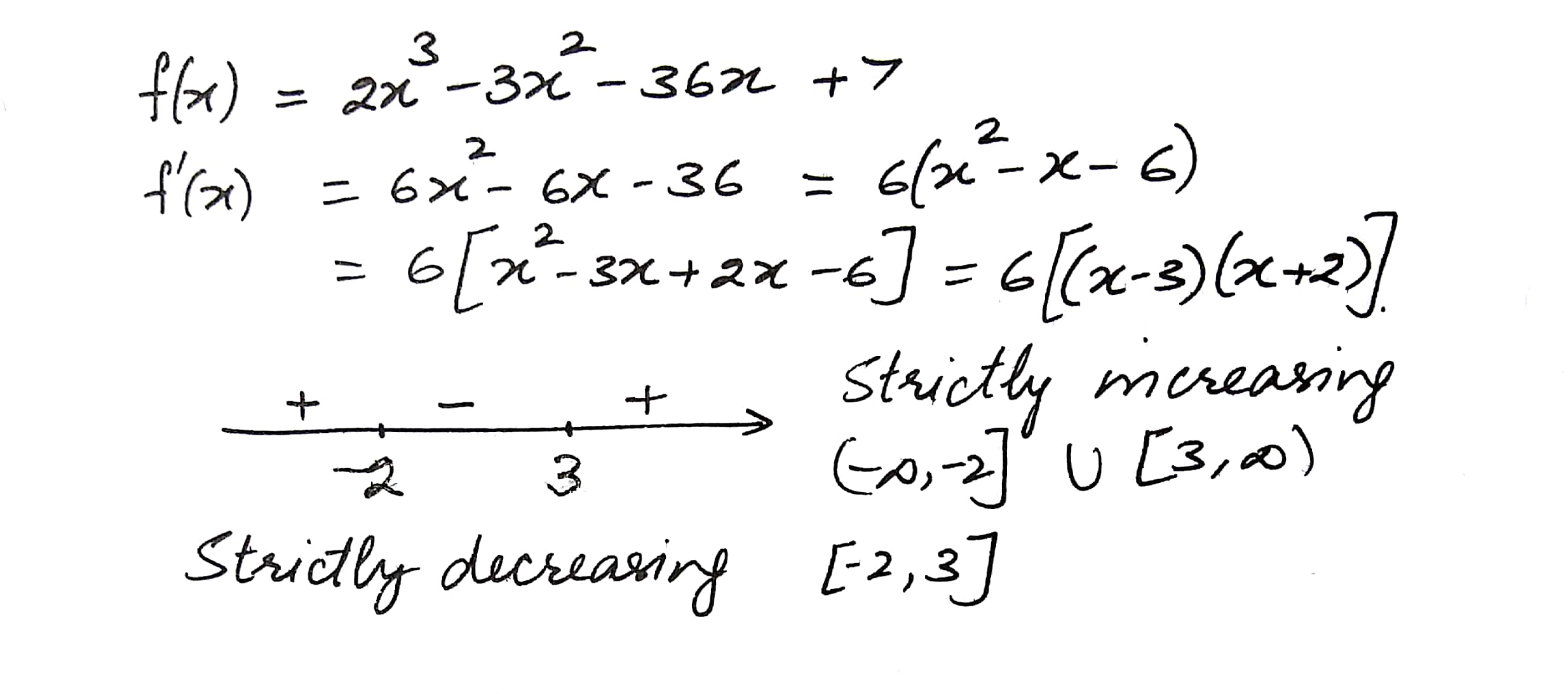5728

Mathematics Integration Level: Misc Level

Find the interval in which the following function is strictly increasing or strictly decreasing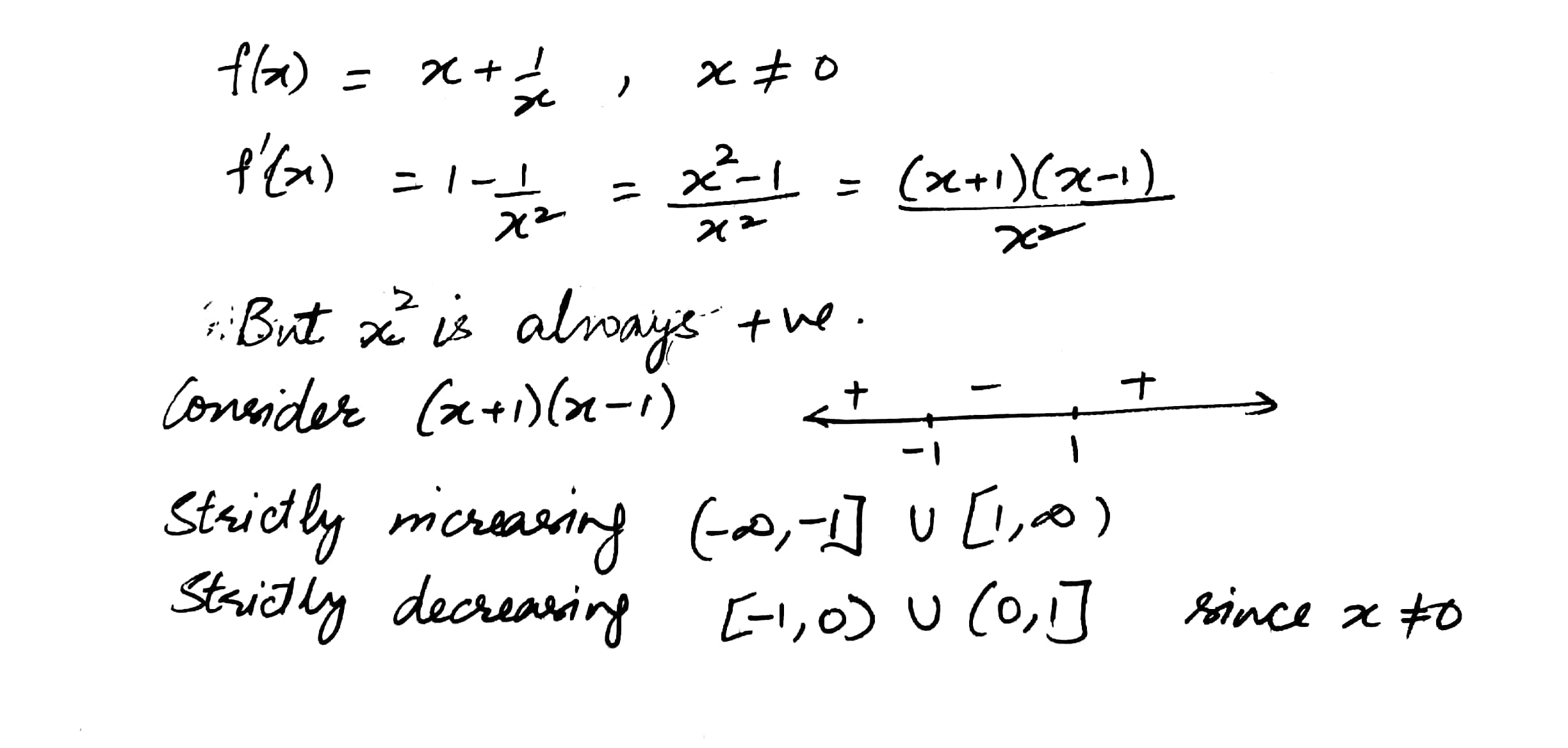5729

Mathematics Integration Level: Misc Level

Find the interval in which the following function is strictly increasing or strictly decreasing

f(x) = x4 - 4x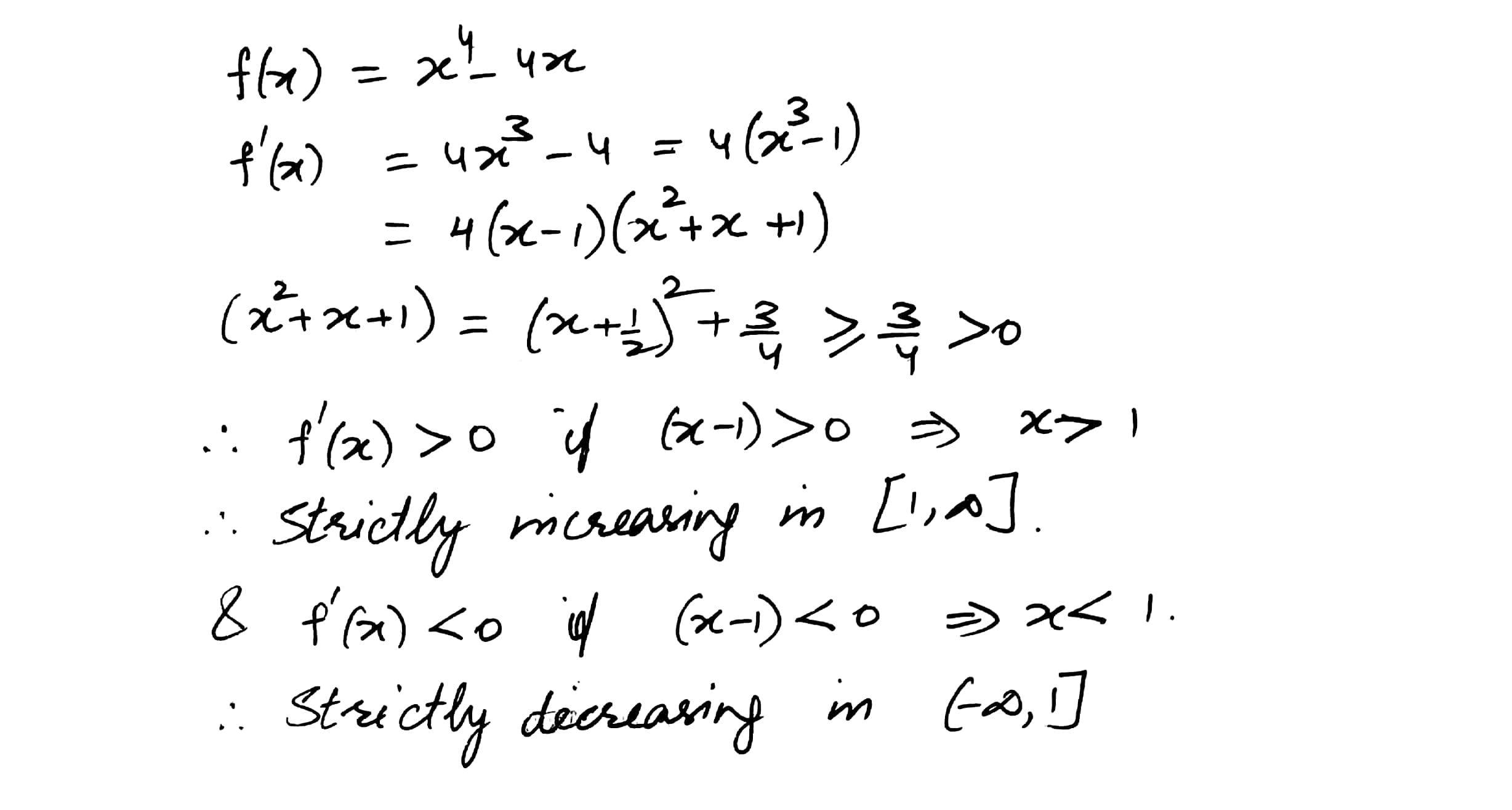Displaying 1-10 of 11 results.